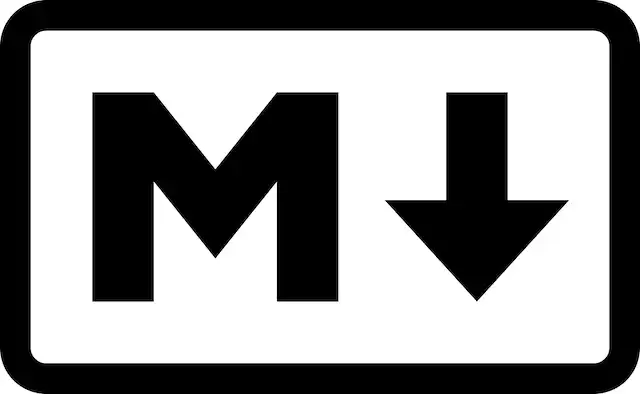# Markdown 介绍

Markdown 是一种轻量级标记语言，创始人为约翰·格鲁伯（John Gruber）。

# 更好的阅读本文

1. 在类似 `#` `1.` `*` 等前置命令后面都要加个空格使其生效。
2. 每个小标题下面先是展示语法的效果（有些片段因为重复，可能会被省略），然后在下面的代码框中是具体的实现代码。中间可能还会穿插着有关代码的说明和提示。
3. `example` 是示例文本，你可以用任意文本替换。但这不代表示例文本都是用 `example` 表示，为了可读性会使用其他文本，具体情况可关注代码框中的实现。
4. 缩进在文中代表一个 `Tab` 或四个 `Space` 。在 `soft tab` 的情况下，此两者是等价的。后面类似的情况这条规则都适用。

# 标题

 ``````1 `````` ``# 标题``

## 一级标题

 ``````1 `````` ``# 一级标题``

 ``````1 2 `````` ``````一级标题 =======``````

## 二级标题

 ``````1 `````` ``## 二级标题``

 ``````1 2 `````` ``````二级标题 -------``````

## 三级标题

 ``````1 `````` ``### 三级标题``

## 四级标题

 ``````1 `````` ``#### 四级标题``

## 五级标题

 ``````1 `````` ``##### 五级标题``

## 六级标题

 ``````1 `````` ``###### 六级标题``

 ``````1 `````` ``[TOC]``

# 列表

## 有序列表

1. 1
2. 2
3. 3

 ``````1 2 3 `````` ``````1. 1 2. 2 3. 3``````

 ``````1 2 3 `````` ``````2. 1 8. 2 4. 3``````

## 无序列表

• 1
• 2
• 3

 ``````1 2 3 `````` ``````* 1 * 2 * 3``````

## 多行列表

1. example

example

2. example

example

 ``````1 2 3 4 5 6 7 `````` ``````1. example example 2. example example``````

## 列表内引用

• example

example

example

 ``````1 2 3 4 5 `````` ``````* example > example > > example``````

# 代码标记

 ``````1 `````` ```printf()```

 ``````1 2 3 `````` ``````printf() printf() printf()``````
 ``````1 2 3 4 5 `````` ````````` printf() printf() printf() `````````

 ``````1 2 3 `````` ``````　　　　printf() 　　　　printf() 　　　　printf()``````

# 引用

## 普通引用

 ``````1 `````` ``> 这里是引用``

1. example
2. example
 ``````1 2 3 `````` ``````> # 标题 > 1. example > 2. example``````

## 嵌套引用

example

example

example

example

 ``````1 2 3 4 5 6 7 `````` ``````> example > > > example > > > > example > > example``````

# 图片与链接

## 图片

### 图片行内式``````1 `````` ``[图片](/images/avatar.webp "title")``

### 图片参考式``````1 2 3 `````` ``````![图片] [pid] [pid]: /images/avatar.webp "title"``````

## 链接

### 自动链接

https://www.sulinehk.com/

 ``````1 `````` ````

 ``````1 `````` ````

### 链接行内式

 ``````1 `````` ``[链接](https://www.sulinehk.com/ "title")``

### 链接参考式

 ``````1 2 3 `````` ``````[链接] [id] [id]: https://www.sulinehk.com/ "title"``````
• 其中，`title` 为鼠标指向 `链接``图片` 时出现的文字。
• 第二行代码可出现在 Markdown 文档的任意位置，`id` 为任意标识符，引导至第二行代码。
• 在一个文档里面需大量使用图片或链接时，建议使用参考式，这样更易于管理和更新。

# 粗体与斜体

## 粗体

 ``````1 2 `````` ``````**粗体** __粗体__``````

## 斜体

 ``````1 2 `````` ``````*斜体* _斜体_``````

# 表格

Table1Table2Table3
a1one
b2two
c3three
 ``````1 2 3 4 5 `````` ``````| Table1 | Table2 | Table3 | | ------ |:-------:| --------:| | a | 1 | one | | b | 2 | two | | c | 3 | three |``````

# 分隔线

 ``````1 2 3 4 5 `````` ``````*** ***** * * * --- ___``````

# 删除线

example

 ``````1 `````` ``~~example~~``

# 反斜杠

*

 ``````1 `````` ``\需要转义的符号（如*）``

 `````` 1 2 3 4 5 6 7 8 9 10 11 12 `````` ``````\ 反斜线 ` 反引号 * 星号 _ 底线 {} 花括号 [] 方括号 () 括弧 # 井字号 + 加号 - 减号 . 英文句点 ! 惊叹号``````

# 复选框列表（github 特有)

• `[x]` `[ ]` 分别表示选中和未选中
• `[x]` `[ ]` 后有个空格
• `[ ]` 中有个空格

• [x] Python

• [x] Go

• [ ] Lisp

• [ ] Ruby

 ``````1 2 3 4 `````` ``````* [x] Python * [x] Go * [ ] Lisp * [ ] Ruby``````

Markdown 编写规范